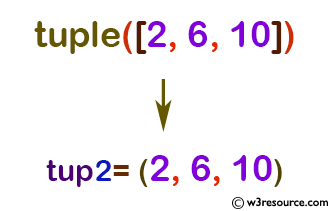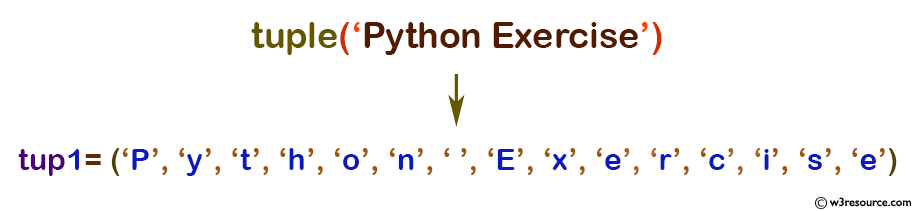# Python: tuple() function

## tuple() function

The tuple() function is used to create a tuple in Python. Iterable may be a sequence, a container that supports iteration, or an iterator object.

Version

(Python 3.2.5)

Syntax:

```tuple([iterable])
```

Parameter:

Name Description Required /
Optional
iterable A sequence, collection or an iterator object. Required.

Example: Python tuple() function

``````tup1 = tuple()
print('tup1=', tup1)

# creating a tuple from a list
tup2 = tuple([2, 6, 10])
print('tup2=', tup2)

# creating a tuple from a string
tup1 = tuple('Python Exercise')
print('tup1=',tup1)

# creating a tuple from a dictionary
tup1 = tuple({3: 'three', 4: 'four'})
print('tup1=',tup1)
``````

Output:

```tup1= ()
tup2= (2, 6, 10)
tup1= ('P', 'y', 't', 'h', 'o', 'n', ' ', 'E', 'x', 'e', 'r', 'c', 'i', 's', 'e')
tup1= (3, 4)
```

Pictorial Presentation:Pictorial Presentation:Python Code Editor:

Previous: sum()
Next: type()

Test your Python skills with w3resource's quiz

﻿

## Python: Tips of the Day

How do I check if a list is empty?

For example, if passed the following:

```a = []
if not a:
print("List is empty")
```

Ref: https://bit.ly/2A4JXx9